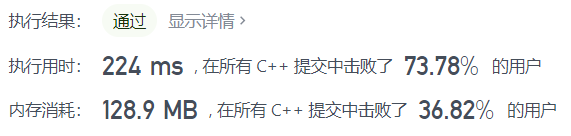# leedcode 239. 滑动窗口最大值(单调队列)

## 题目描述

---------------               -----
[1  3  -1] -3  5  3  6  7       3
1 [3  -1  -3] 5  3  6  7       3
1  3 [-1  -3  5] 3  6  7       5
1  3  -1 [-3  5  3] 6  7       5
1  3  -1  -3 [5  3  6] 7       6
1  3  -1  -3  5 [3  6  7]      7

1 <= nums.length <= 105
-104 <= nums[i] <= 104
1 <= k <= nums.length

[1,3,1,2,0,5] 3

[3,2,2,5]

[3,3,2,5]

## 题解

• 双端单调队列 经典题
• 想到了单调队列，具体怎么用，为什么对它有效不清楚。看懂了思路，能写出来。
• 窗口一旦滑倒后面的数，后面的数一旦入队列，那么前面比当前这个数小的数就不可能是最大值了。比如  [1 3 -1 2 0 5 ]，k=，一开始窗口 [1 3 -1 2]，最大值是3，队列为 [ 3，2] ，为什么不是 [ 1 3 -1 2 ] 呢？因为窗口一旦滑到后面，有 3 在，就不可能 1 最大，1 没必要存在于队列中，同理，有 2 在 -1 后面，又比 -1 大，-1不可能是最大的数，-1也没必要存在队列中。这种特性就适合用单调队列。
class Solution {
public:
vector<int> maxSlidingWindow(vector<int>& nums, int k) {
deque<int>q;//双端单调队列 队列从大到小
vector<int>ans;
int n=nums.size();
for(int i=0;i<k;i++){
while(!q.empty()&&q.back()<nums[i]){//如果队尾比当前数小
q.pop_back();//把队尾的数删掉
}
q.push_back(nums[i]);//把当前数放入队尾

}
ans.push_back(q.front());//记录最大值 最大值总在队头
for(int j=k;j<n;j++){//指向滑动窗口最后一个数的后一个数
int i=j-k;//指向滑动窗口的第一个数
if(q.front()==nums[i]){//如果窗口向右滑动 这个数又是最大值
q.pop_front();//那就删除
}
while(!q.empty()&&q.back()<nums[j]){//同上 维护单调队列
q.pop_back();
}
q.push_back(nums[j]);//把当前数放入队尾
ans.push_back(q.front());
}
return ans;
}
};• 其它解法，优先队列
• 没考虑过
class Solution {
public:
vector maxSlidingWindow(vector& nums, int k) {
int n = nums.size();
priority_queue> q;
for (int i = 0; i < k; ++i) {
q.emplace(nums[i], i);
}
vector ans = {q.top().first};
for (int i = k; i < n; ++i) {
q.emplace(nums[i], i);
while (q.top().second <= i - k) {
q.pop();
}
ans.push_back(q.top().first);
}
return ans;
}
};

posted on 2021-09-16 17:51  蔡军帅  阅读(10)  评论(0编辑  收藏  举报